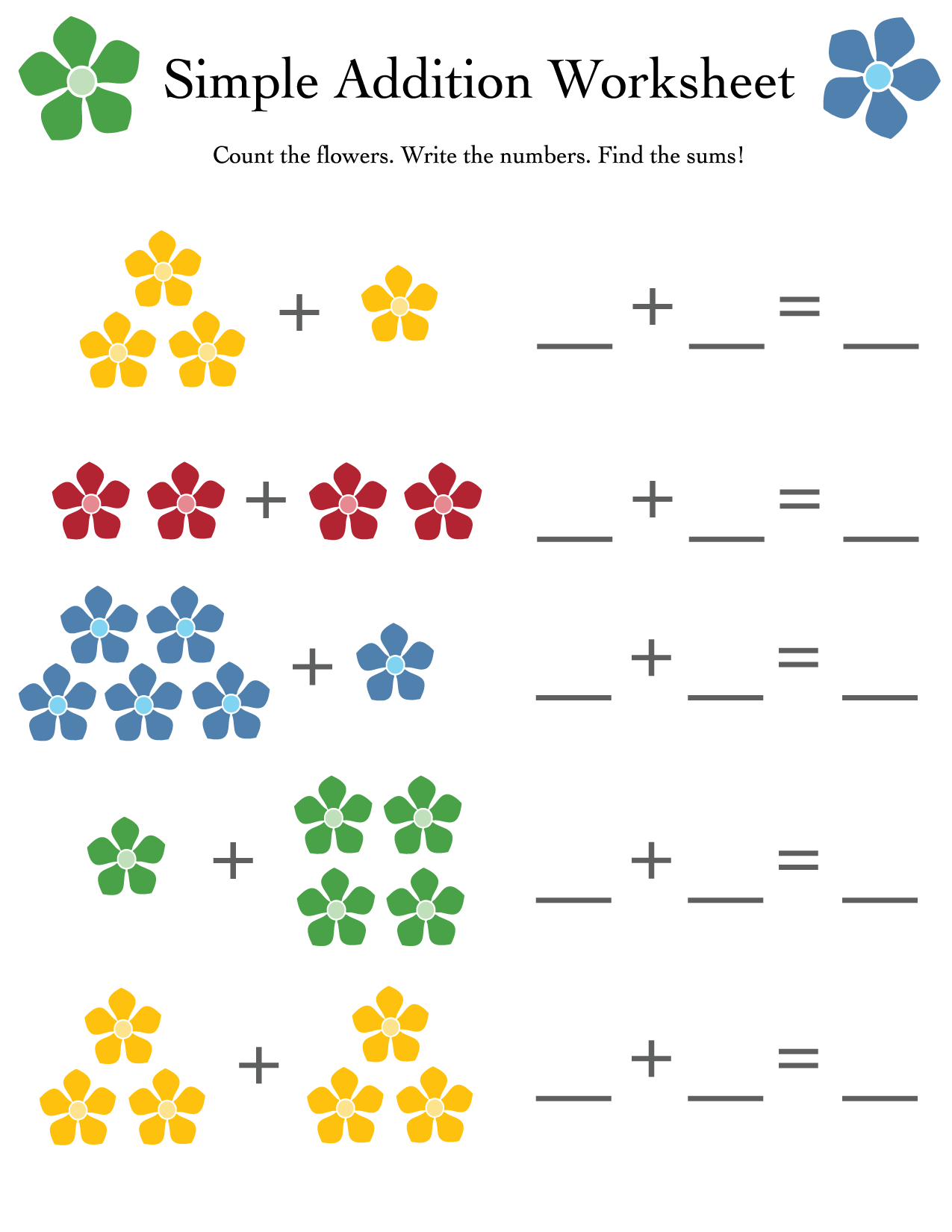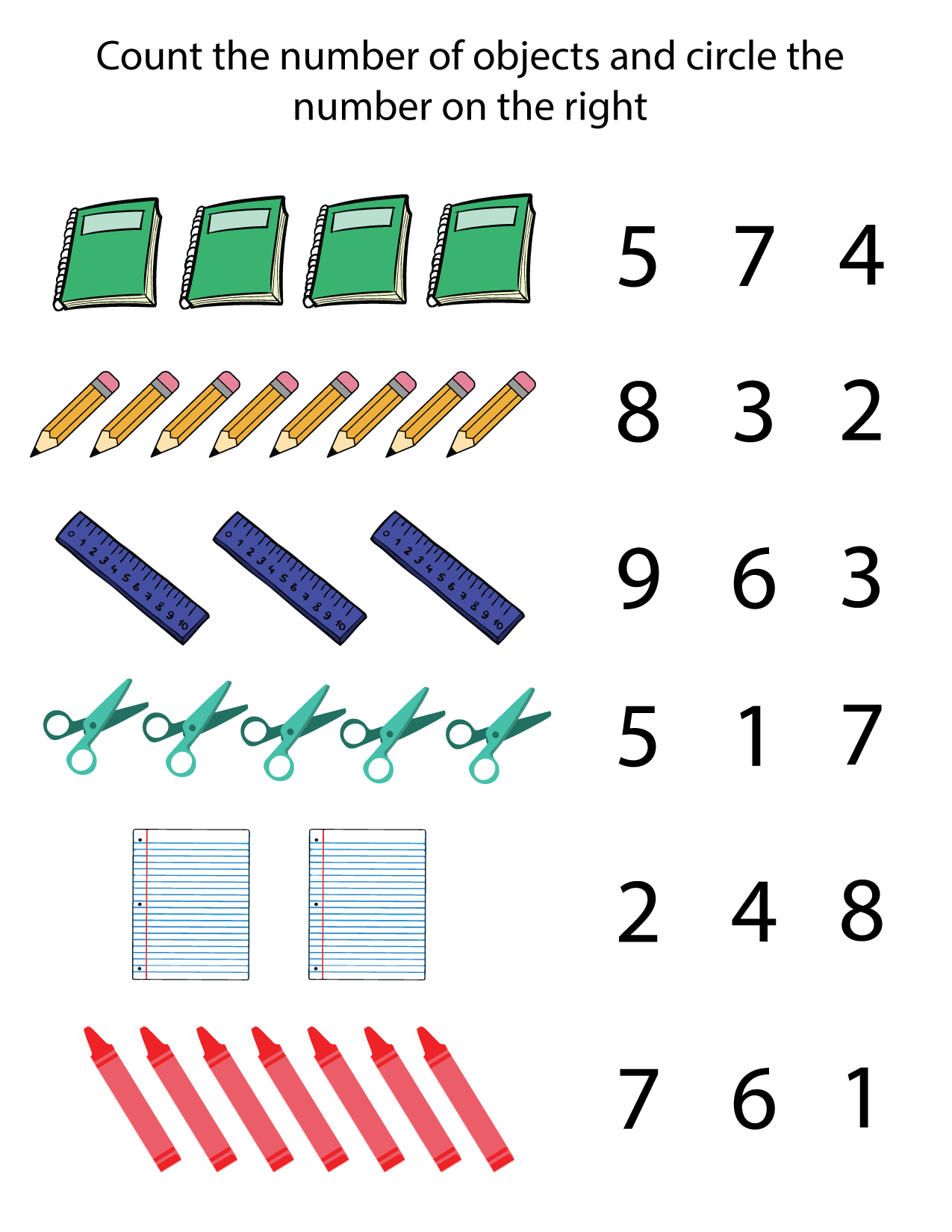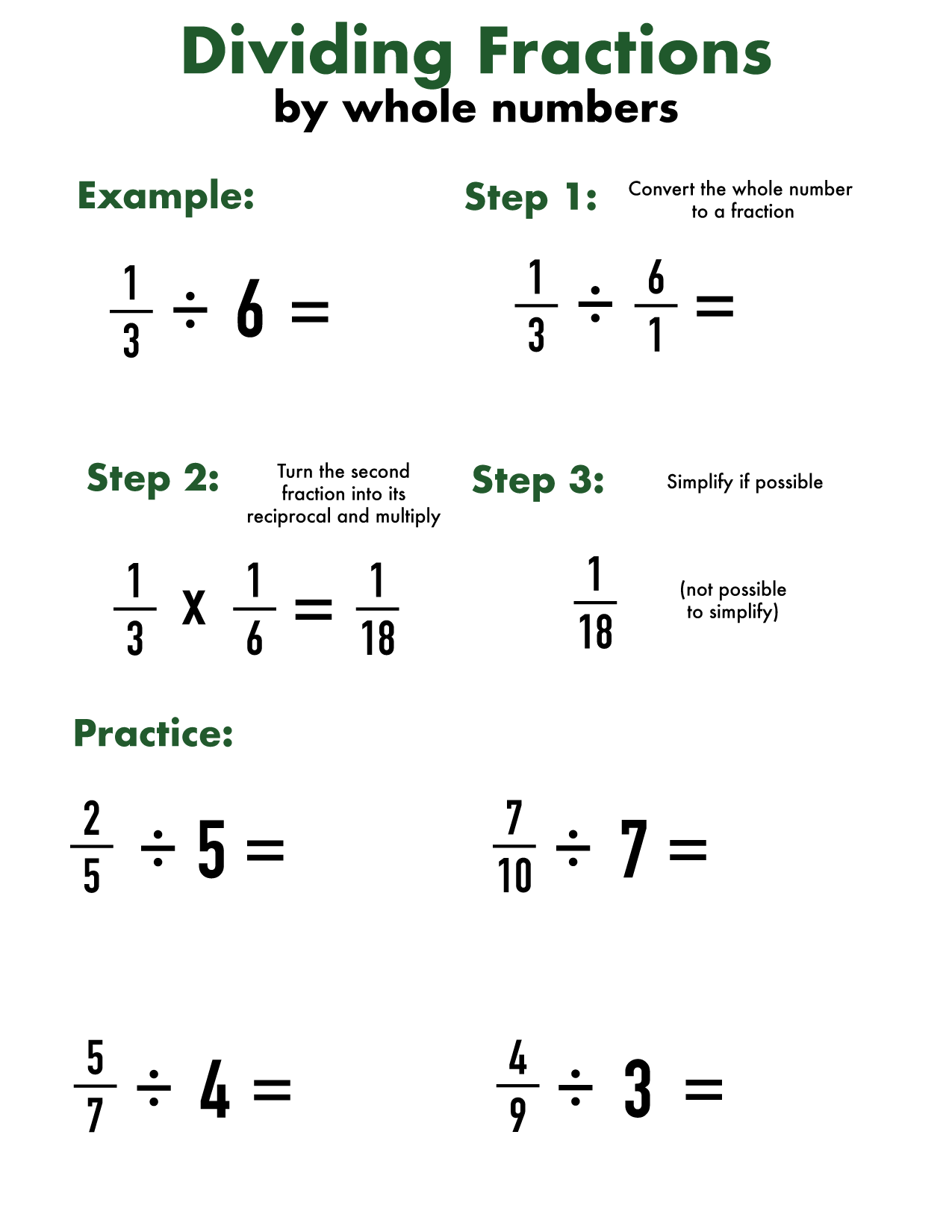## Printable dividing fractions by whole numbers worksheet (PDF)

Dividing fractions is the most difficult of functions involving fractions, but it’s not that hard once you get the hang of it! In this post, we will both give...## Printable multiplying fractions worksheets (by whole numbers) in PDF

Multiplying fractions is not that hard once you get used to it. In this post, we will get kids used to multiplying fractions by teaching them how it works...## Printable adding and subtracting fractions worksheets (with different or unlike denominators)

After students learn what fractions are, the next step is learning how to add and subtract them. Fractions with the same (or ‘like’) denominators are easier to add and...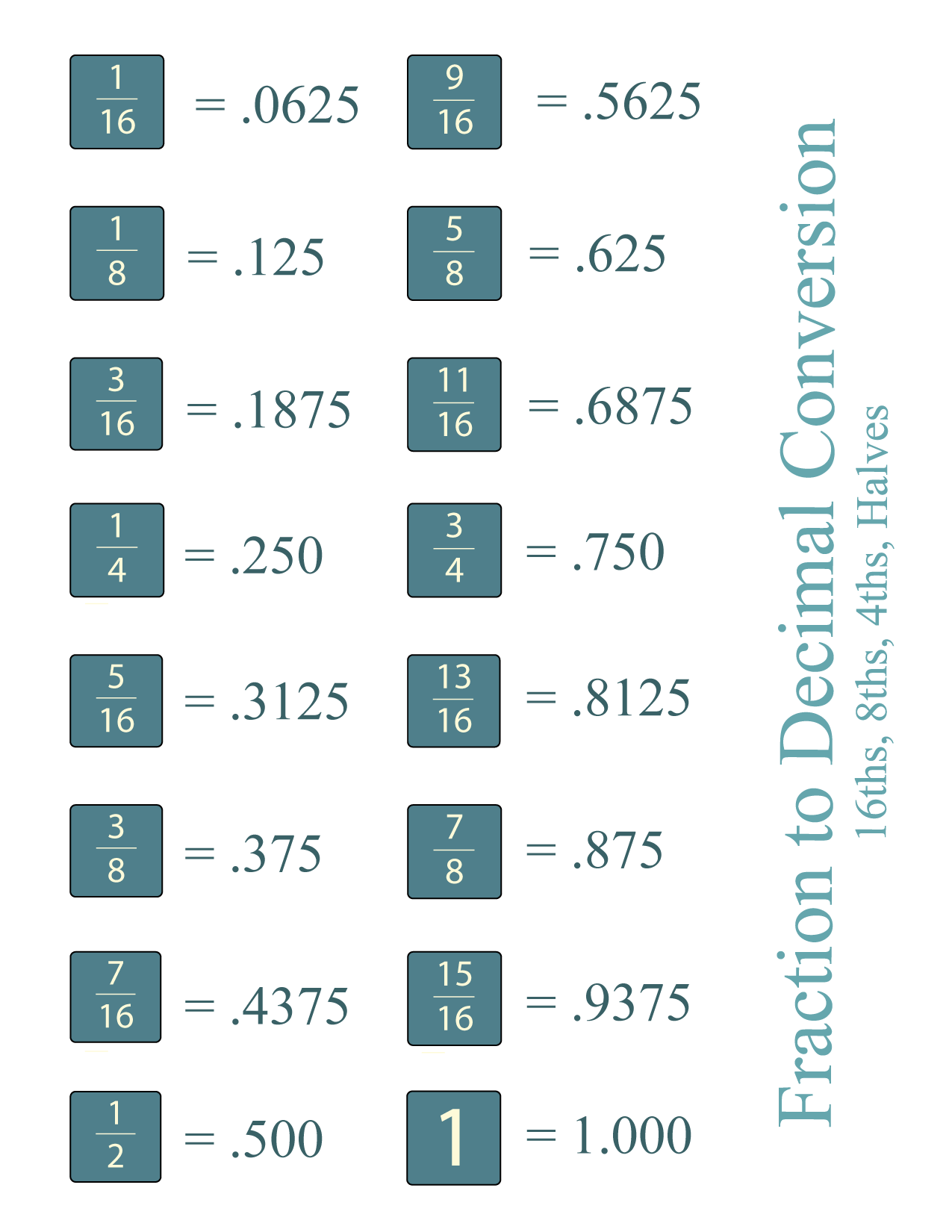## Printable fraction to decimal conversion tables (PDF)

Fractions and decimals are two ways of expressing parts of a whole, but it can be hard (especially for students) to remember how they relate to each other. In...## Free and printable 4th grade math worksheets (pdf)

After learning all the 3rd grade math, it’s time to move onto 4th grade math! If you want additional worksheets, check out our post here. In past worksheets, students...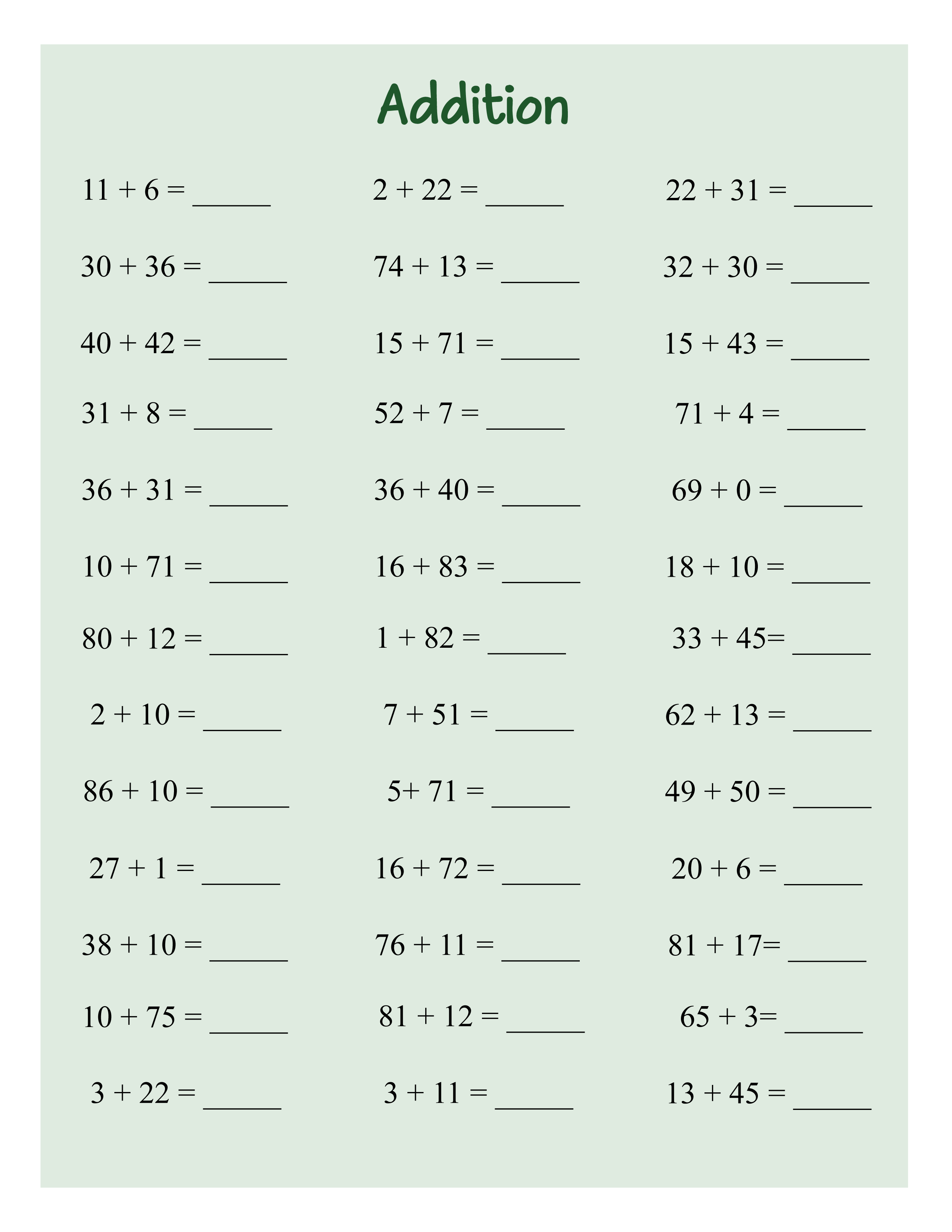## Free printable 3rd grade math worksheets

3rd grade is when students get even more involved with math, specifically starting to work more on multiplication and division skills. In this post, we have an addition worksheet,...Getting kids used to math in school can be difficult, and it’s also hard to find ways to make it fun! In this post, we have free math sheets...